• 而且也可以方便地将它作为绘图控件，嵌入GUI应用程序中。 它的文档相当完备，并且Gallery页面中有上百幅缩略图，打开之后都有源程序。因此如果你需要绘制某种类型的图，只需要在这个页面中浏览/复制/粘贴一

Python图表绘制：matplotlib绘图库入门

matplotlib 是python最著名的绘图库，它提供了一整套和matlab相似的命令API，十分适合交互式地行制图。而且也可以方便地将它作为绘图控件，嵌入GUI应用程序中。

它的文档相当完备，并且Gallery页面中有上百幅缩略图，打开之后都有源程序。因此如果你需要绘制某种类型的图，只需要在这个页面中浏览/复制/粘贴一下，基本上都能搞定。

在Linux下比较著名的数据图工具还有gnuplot，这个是免费的，Python有一个包可以调用gnuplot，但是语法比较不习惯，而且画图质量不高。

而 Matplotlib则比较强：Matlab的语法、python语言、latex的画图质量（还可以使用内嵌的latex引擎绘制的数学公式）。

Matplotlib.pyplot快速绘图

快速绘图和面向对象方式绘图

matplotlib实际上是一套面向对象的绘图库，它所绘制的图表中的每个绘图元素，例如线条Line2D、文字Text、刻度等在内存中都有一个对象与之对应。

为了方便快速绘图matplotlib通过pyplot模块提供了一套和MATLAB类似的绘图API，将众多绘图对象所构成的复杂结构隐藏在这套API内部。我们只需要调用pyplot模块所提供的函数就可以实现快速绘图以及设置图表的各种细节。pyplot模块虽然用法简单，但不适合在较大的应用程序中使用。

为了将面向对象的绘图库包装成只使用函数的调用接口，pyplot模块的内部保存了当前图表以及当前子图等信息。当前的图表和子图可以使用plt.gcf()和plt.gca()获得，分别表示"Get Current Figure"和"Get Current Axes"。在pyplot模块中，许多函数都是对当前的Figure或Axes对象进行处理，比如说：

plt.plot()实际上会通过plt.gca()获得当前的Axes对象ax，然后再调用ax.plot()方法实现真正的绘图。

可以在Ipython中输入类似"plt.plot??"的命令查看pyplot模块的函数是如何对各种绘图对象进行包装的。

配置属性

matplotlib所绘制的图表的每个组成部分都和一个对象对应，我们可以通过调用这些对象的属性设置方法set_*()或者pyplot模块的属性设置函数setp()设置它们的属性值。

因为matplotlib实际上是一套面向对象的绘图库，因此也可以直接获取对象的属性

配置文件

绘制一幅图需要对许多对象的属性进行配置，例如颜色、字体、线型等等。我们在绘图时，并没有逐一对这些属性进行配置，许多都直接采用了matplotlib的缺省配置。

matplotlib将这些缺省配置保存在一个名为“matplotlibrc”的配置文件中，通过修改配置文件，我们可以修改图表的缺省样式。配置文件的读入可以使用rc_params()，它返回一个配置字典；在matplotlib模块载入时会调用rc_params()，并把得到的配置字典保存到rcParams变量中；matplotlib将使用rcParams字典中的配置进行绘图；用户可以直接修改此字典中的配置，所做的改变会反映到此后创建的绘图元素。

绘制多子图（快速绘图）

Matplotlib 里的常用类的包含关系为 Figure -> Axes -> (Line2D, Text, etc.)一个Figure对象可以包含多个子图(Axes)，在matplotlib中用Axes对象表示一个绘图区域，可以理解为子图。

可以使用subplot()快速绘制包含多个子图的图表，它的调用形式如下：
subplot(numRows, numCols, plotNum)

subplot将整个绘图区域等分为numRows行* numCols列个子区域，然后按照从左到右，从上到下的顺序对每个子区域进行编号，左上的子区域的编号为1。如果numRows，numCols和plotNum这三个数都小于10的话，可以把它们缩写为一个整数，例如subplot(323)和subplot(3,2,3)是相同的。subplot在plotNum指定的区域中创建一个轴对象。如果新创建的轴和之前创建的轴重叠的话，之前的轴将被删除。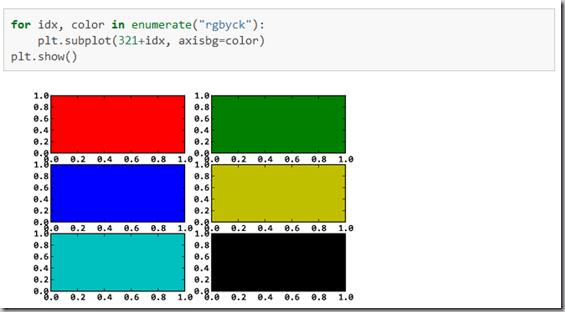subplot()返回它所创建的Axes对象，我们可以将它用变量保存起来，然后用sca()交替让它们成为当前Axes对象，并调用plot()在其中绘图。

绘制多图表（快速绘图）

如果需要同时绘制多幅图表，可以给figure()传递一个整数参数指定Figure对象的序号，如果序号所指定的Figure对象已经存在，将不创建新的对象，而只是让它成为当前的Figure对象。
import numpy as np
import matplotlib.pyplot as plt
plt.figure(1) # 创建图表1
plt.figure(2) # 创建图表2
ax1 = plt.subplot(211) # 在图表2中创建子图1
ax2 = plt.subplot(212) # 在图表2中创建子图2
x = np.linspace(0, 3, 100)
for i in xrange(5):
plt.figure(1)  #❶ # 选择图表1
plt.plot(x, np.exp(i*x/3))
plt.sca(ax1)   #❷ # 选择图表2的子图1
plt.plot(x, np.sin(i*x))
plt.sca(ax2)  # 选择图表2的子图2
plt.plot(x, np.cos(i*x))
plt.show()在图表中显示中文

matplotlib的缺省配置文件中所使用的字体无法正确显示中文。为了让图表能正确显示中文，可以有几种解决方案。
在程序中直接指定字体。在程序开头修改配置字典rcParams。修改配置文件。

面向对象画图

matplotlib API包含有三层，Artist层处理所有的高层结构，例如处理图表、文字和曲线等的绘制和布局。通常我们只和Artist打交道，而不需要关心底层的绘制细节。

直接使用Artists创建图表的标准流程如下：
创建Figure对象用Figure对象创建一个或者多个Axes或者Subplot对象调用Axies等对象的方法创建各种简单类型的Artistsimport matplotlib.pyplot as plt
X1 = range(0, 50)
Y1 = [num**2 for num in X1] # y = x^2
X2 = [0, 1]
Y2 = [0, 1]  # y = x
Fig = plt.figure(figsize=(8,4))                      # Create a figure' instance
Ax = Fig.add_subplot(111)               # Create a axes' instance in the figure
Ax.plot(X1, Y1, X2, Y2)                 # Create a Line2D instance in the axes
Fig.show()
Fig.savefig("test.pdf")

参考：

《Python科学计算》(Numpy视频) matplotlib-绘制精美的图表(快速绘图)(面向对象绘图)（深入浅出适合系统学习）

什么是 Matplotlib （主要讲面向对象绘图，对于新手可能有点乱）

Matplotlib.pylab快速绘图

matplotlib还提供了一个名为pylab的模块，其中包括了许多NumPy和pyplot模块中常用的函数，方便用户快速进行计算和绘图，十分适合在IPython交互式环境中使用。这里使用下面的方式载入pylab模块：
>>> import pylab as pl

1 安装numpy和matplotlib

>>> import numpy
>>> numpy.__version__

>>> import matplotlib
>>> matplotlib.__version__

2 两种常用图类型：Line and scatter plots(使用plot()命令), histogram(使用hist()命令)

2.1 折线图&散点图 Line and scatter plots

2.1.1 折线图 Line plots(关联一组x和y值的直线)
import numpy as np
import pylab as pl
x = [1, 2, 3, 4, 5]# Make an array of x values
y = [1, 4, 9, 16, 25]# Make an array of y values for each x value
pl.plot(x, y)# use pylab to plot x and y
pl.show()# show the plot on the screen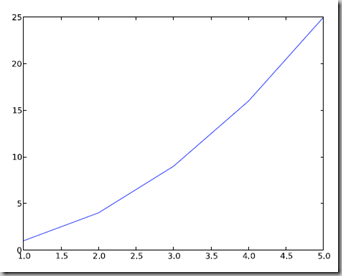2.1.2 散点图 Scatter plots

把pl.plot(x, y)改成pl.plot(x, y, 'o')即可，下图的蓝色版本

2.2  美化 Making things look pretty

2.2.1 线条颜色 Changing the line color

红色：把pl.plot(x, y, 'o')改成pl.plot(x, y, ’or’)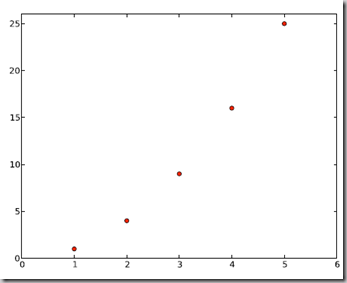2.2.2 线条样式 Changing the line style

虚线:plot(x,y, '--')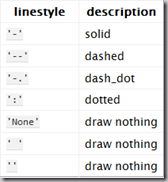2.2.3 marker样式 Changing the marker style

蓝色星型markers：plot(x,y, ’b*’)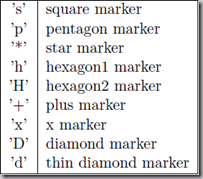2.2.4 图和轴标题以及轴坐标限度 Plot and axis titles and limits
import numpy as np
import pylab as pl
x = [1, 2, 3, 4, 5]# Make an array of x values
y = [1, 4, 9, 16, 25]# Make an array of y values for each x value
pl.plot(x, y)# use pylab to plot x and y
pl.title(’Plot of y vs. x’)# give plot a title
pl.xlabel(’x axis’)# make axis labels
pl.ylabel(’y axis’)
pl.xlim(0.0, 7.0)# set axis limits
pl.ylim(0.0, 30.)
pl.show()# show the plot on the screen2.2.5 在一个坐标系上绘制多个图 Plotting more than one plot on the same set of axes

做法是很直接的，依次作图即可:
import numpy as np
import pylab as pl
x1 = [1, 2, 3, 4, 5]# Make x, y arrays for each graph
y1 = [1, 4, 9, 16, 25]
x2 = [1, 2, 4, 6, 8]
y2 = [2, 4, 8, 12, 16]
pl.plot(x1, y1, ’r’)# use pylab to plot x and y
pl.plot(x2, y2, ’g’)
pl.title(’Plot of y vs. x’)# give plot a title
pl.xlabel(’x axis’)# make axis labels
pl.ylabel(’y axis’)
pl.xlim(0.0, 9.0)# set axis limits
pl.ylim(0.0, 30.)
pl.show()# show the plot on the screen2.2.6  图例 Figure legends

pl.legend((plot1, plot2), (’label1, label2’), 'best’, numpoints=1)

其中第三个参数表示图例放置的位置:'best’‘upper right’, ‘upper left’, ‘center’, ‘lower left’, ‘lower right’.

如果在当前figure里plot的时候已经指定了label，如plt.plot(x,z,label="$cos(x^2)$")，直接调用plt.legend()就可以了哦。
import numpy as np
import pylab as pl
x1 = [1, 2, 3, 4, 5]# Make x, y arrays for each graph
y1 = [1, 4, 9, 16, 25]
x2 = [1, 2, 4, 6, 8]
y2 = [2, 4, 8, 12, 16]
plot1 = pl.plot(x1, y1, ’r’)# use pylab to plot x and y : Give your plots names
plot2 = pl.plot(x2, y2, ’go’)
pl.title(’Plot of y vs. x’)# give plot a title
pl.xlabel(’x axis’)# make axis labels
pl.ylabel(’y axis’)
pl.xlim(0.0, 9.0)# set axis limits
pl.ylim(0.0, 30.)
pl.legend([plot1, plot2], (’red line’, ’green circles’), ’best’, numpoints=1)# make legend
pl.show()# show the plot on the screen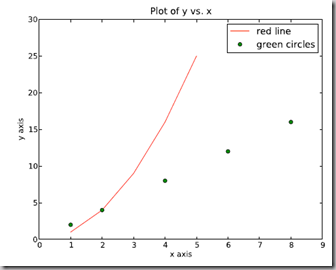2.3 直方图 Histograms
import numpy as np
import pylab as pl
# make an array of random numbers with a gaussian distribution with
# mean = 5.0
# rms = 3.0
# number of points = 1000
data = np.random.normal(5.0, 3.0, 1000)
# make a histogram of the data array
pl.hist(data)
# make plot labels
pl.xlabel(’data’)
pl.show()

如果不想要黑色轮廓可以改为pl.hist(data, histtype=’stepfilled’)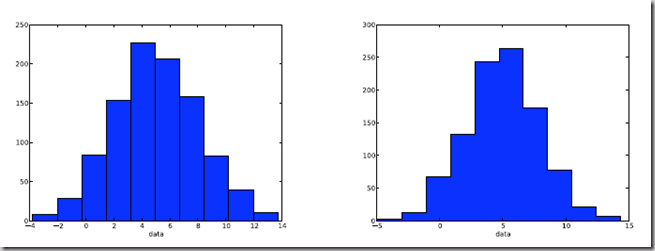2.3.1 自定义直方图bin宽度 Setting the width of the histogram bins manually

增加这两行

bins = np.arange(-5., 16., 1.) #浮点数版本的range
pl.hist(data, bins, histtype=’stepfilled’)3 同一画板上绘制多幅子图 Plotting more than one axis per canvas

如果需要同时绘制多幅图表的话，可以是给figure传递一个整数参数指定图标的序号，如果所指定
序号的绘图对象已经存在的话，将不创建新的对象，而只是让它成为当前绘图对象。

fig1 = pl.figure(1)
pl.subplot(211)
subplot(211)把绘图区域等分为2行*1列共两个区域, 然后在区域1(上区域)中创建一个轴对象. pl.subplot(212)在区域2(下区域)创建一个轴对象。You can play around with plotting a variety of layouts. For example, Fig. 11 is created using the following commands:

f1 = pl.figure(1)
pl.subplot(221)
pl.subplot(222)
pl.subplot(212)当绘图对象中有多个轴的时候，可以通过工具栏中的Configure Subplots按钮，交互式地调节轴之间的间距和轴与边框之间的距离。如果希望在程序中调节的话，可以调用subplots_adjust函数，它有left, right, bottom, top, wspace, hspace等几个关键字参数，这些参数的值都是0到1之间的小数，它们是以绘图区域的宽高为1进行正规化之后的坐标或者长度。

pl.subplots_adjust(left=0.08, right=0.95, wspace=0.25, hspace=0.45)

4 绘制文件中的数据Plotting data contained in files

4.1 从Ascii文件中读取数据 Reading data from ascii files

读取文件的方法很多，这里只介绍一种简单的方法，更多的可以参考官方文档和NumPy快速处理数据(文件存取)。

numpy的loadtxt方法可以直接读取如下文本数据到numpy二维数组

**********************************************

# fakedata.txt
0 0
1 1
2 4
3 9
4 16
5 25
6 36
7 49
8 64
9 81
0 0
1 1
2 4
3 9
4 16
5 25
6 36
7 49
8 64
9 81

**********************************************
import numpy as np
import pylab as pl
# Use numpy to load the data contained in the file
# ’fakedata.txt’ into a 2-D array called data
data = np.loadtxt(’fakedata.txt’)
# plot the first column as x, and second column as y
pl.plot(data[:,0], data[:,1], ’ro’)
pl.xlabel(’x’)
pl.ylabel(’y’)
pl.xlim(0.0, 10.)
pl.show()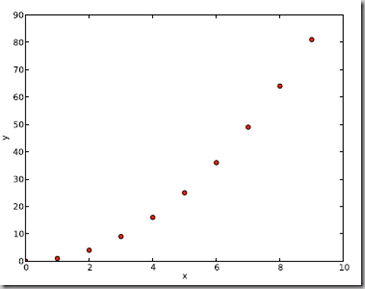4.2 写入数据到文件 Writing data to a text file

写文件的方法也很多，这里只介绍一种可用的写入文本文件的方法，更多的可以参考官方文档。
import numpy as np
# Let’s make 2 arrays (x, y) which we will write to a file
# x is an array containing numbers 0 to 10, with intervals of 1
x = np.arange(0.0, 10., 1.)
# y is an array containing the values in x, squared
y = x*x
print ’x = ’, x
print ’y = ’, y
# Now open a file to write the data to
# ’w’ means open for ’writing’
file = open(’testdata.txt’, ’w’)
# loop over each line you want to write to file
for i in range(len(x)):
# make a string for each line you want to write
# ’\t’ means ’tab’
# ’\n’ means ’newline’
# ’str()’ means you are converting the quantity in brackets to a string type
txt = str(x[i]) + ’\t’ + str(y[i]) + ’ \n’
# write the txt to the file
file.write(txt)
# Close your file
file.close()

这部分是翻译自：Python Plotting Beginners Guide

对LaTeX数学公式的支持

Matlplotlib对LaTeX有一定的支持，如果记得使用raw字符串语法会很自然：

xlabel(r"$\frac{x^2}{y^4}$")

在matplotlib里面，可以使用LaTex的命令来编辑公式，只需要在字符串前面加一个“r”即可

Here is a simple example:

# plain text
plt.title('alpha > beta')

produces “alpha > beta”.

Whereas this:

# math text
plt.title(r'$\alpha > \beta$')

produces "".

这里给大家看一个简单的例子。

import matplotlib.pyplot as plt
x = arange(1,1000,1)
r = -2
c = 5
y = [5*(a**r) for a in x]

fig = plt.figure()
ax = fig.add_subplot(111)
ax.loglog(x,y,label = r"$y = \frac{1}{2\sigma_1^2}, c=5,\sigma_1=-2$")
ax.legend()
ax.set_xlabel(r"x")
ax.set_ylabel(r"y")

程序执行结果如图3所示，这实际上是一个power-law的例子，有兴趣的朋友可以继续google之。

再看一个《用Python做科学计算》中的简单例子，下面的两行程序通过调用plot函数在当前的绘图对象中进行绘图：

plt.plot(x,y,label="$sin(x)$",color="red",linewidth=2)
plt.plot(x,z,"b--",label="$cos(x^2)$")

plot函数的调用方式很灵活，第一句将x,y数组传递给plot之后，用关键字参数指定各种属性：
label : 给所绘制的曲线一个名字，此名字在图示(legend)中显示。只要在字符串前后添加"\$"符号，matplotlib就会使用其内嵌的latex引擎绘制的数学公式。color : 指定曲线的颜色linewidth : 指定曲线的宽度

详细的可以参考matplotlib官方教程:

Writing mathematical expressions
Subscripts and superscriptsFractions, binomials and stacked numbersRadicalsFonts
Custom fontsAccentsSymbolsExample
Text rendering With LaTeX
usetex with unicodePostscript optionsPossible hangupsTroubleshooting


展开全文• Python中的数据可视化matplotlib 是python最著名的绘图库，它提供了一整套和matlab相似的命令API，十分适合交互式地进行制图。而且也可以方便地将它作为绘图控件。实战小程序：画出y=x^3的散点图样例代码如下：#...
Python中的数据可视化matplotlib 是python最著名的绘图库，它提供了一整套和matlab相似的命令API，十分适合交互式地进行制图。而且也可以方便地将它作为绘图控件。实战小程序：画出y=x^3的散点图样例代码如下：#coding=utf-8import pylab as y  #引入pylab模块x = y.np.linspace(-10,10,100) #设置x横坐标范围和点数y.plot(x,x*x*x,'or') #生成图像ax = y.gca()ax.spines['right'].set_color('none')ax.spines['top'].set_color('none')ax.xaxis.set_ticks_position('bottom')ax.spines['bottom'].set_position(('data',0))ax.yaxis.set_ticks_position('left')ax.spines['left'].set_position(('data',0))ax.set_yticks([-1000,-500,500,1000])y.xlim(x.min(),x.max() ) #将横坐标设置为x的最大值和最小值y.show() #显示图像import pylab as y程序中引入的pylab属于matplotlib的一个模块，将其名字用y代替，其中包括了许多NumPy和pyplot模块中常用的函数，方便用户快速进行计算和绘图，十分适合在IPython交互式环境中使用。y.np.linspace(-10,100)此为numpy中的一个函数，返回的是等间距的值，numpy.linspace(a,b,c):a指的是开始位置，b表示的是结束位置，c表示产生点的个数（默认为50）举例：>>> np.linspace(2.0,3.0,num=5)array([ 2.,2.25,2.5,2.75,3. ])y.plot(x,'or') #生成图像后面加上"o'表示为散点图'r'可设置颜色为红色，基本上和matlab的操作很像。y.xlim(x.min(),x.max())这条语句使用了xlim函数，将横坐标设置为x的大小结果示例：总结以上就是本文关于Python实战小程序利用matplotlib模块画图代码分享的全部内容，希望对大家有所帮助。感兴趣的朋友可以继续参阅本站其他相关专题。如有不足之处，欢迎留言指出。感谢朋友们对本站的支持！
展开全文• Python中的数据可视化matplotlib 是python最著名的绘图库，它提供了一整套和matlab相似的命令API，十分适合交互式地进行制图。而且也可以方便地将它作为绘图控件。实战小程序：画出y=x^3的散点图样例代码如下：#...
Python中的数据可视化matplotlib 是python最著名的绘图库，它提供了一整套和matlab相似的命令API，十分适合交互式地进行制图。而且也可以方便地将它作为绘图控件。实战小程序：画出y=x^3的散点图样例代码如下：#coding=utf-8import pylab as y  #引入pylab模块x = y.np.linspace(-10, 10, 100) #设置x横坐标范围和点数y.plot(x, x*x*x,'or') #生成图像ax = y.gca()ax.spines['right'].set_color('none')ax.spines['top'].set_color('none')ax.xaxis.set_ticks_position('bottom')ax.spines['bottom'].set_position(('data', 0))ax.yaxis.set_ticks_position('left')ax.spines['left'].set_position(('data', 0))ax.set_yticks([-1000, -500, 500, 1000])y.xlim(x.min() , x.max() ) #将横坐标设置为x的最大值和最小值y.show() #显示图像import pylab as y程序中引入的pylab属于matplotlib的一个模块，将其名字用y代替，其中包括了许多NumPy和pyplot模块中常用的函数，方便用户快速进行计算和绘图，十分适合在IPython交互式环境中使用。y.np.linspace(-10, 10, 100)此为numpy中的一个函数，返回的是等间距的值，numpy.linspace(a,b,c):a指的是开始位置，b表示的是结束位置，c表示产生点的个数（默认为50）举例：>>> np.linspace(2.0, 3.0, num=5)array([ 2. , 2.25, 2.5 , 2.75, 3. ])y.plot(x, x*x*x,'or') #生成图像后面加上"o'表示为散点图'r'可设置颜色为红色，基本上和matlab的操作很像。y.xlim(x.min(), x.max())这条语句使用了xlim函数，将横坐标设置为x的大小结果示例：总结以上就是本文关于Python实战小程序利用matplotlib模块画图代码分享的全部内容，希望对大家有所帮助。感兴趣的朋友可以继续参阅本站其他相关专题。如有不足之处，欢迎留言指出。感谢朋友们对本站的支持！
展开全文• 图片来自 unsplash上篇文章，我们了解到 Matplotlib 是一个风格类似 Matlab 的基于 Python 的绘图库。它提供了一整套和matlab相似的命令API，十分适合交互式地进行制图。而且我们也可以方便地将它作为绘图控件，嵌入...图片来自 unsplash上篇文章，我们了解到 Matplotlib 是一个风格类似 Matlab 的基于 Python 的绘图库。它提供了一整套和matlab相似的命令API，十分适合交互式地进行制图。而且我们也可以方便地将它作为绘图控件，嵌入GUI应用程序中。本文主要走进 Matplotlib 的世界，初步学会绘制图形。1 基础知识在学习绘制之前，先来了解下 Matplotlib 基础概念。1.1 库我们绘制图形主要用到两个库，matplotlib.pyplot和numpy。在编码过程中，这两个库的使用频率较高，而这两个库的名字较长。这难免会给我们带来不便。所以我们一般给其设置别名， 大大减少重复性工作量。具体如下代码：import matplotlib.pyplot as plt   # 导入模块 matplotlib.pyplot，并简写成 pltimport numpy as np                # 导入模块 numpy，并简写成 npnumpy 是 Python 用于数学运算的库，它是在安装 matplotlib 时候顺带安装的。pyplot 是 matplotlib 一个子模块，主要为底层的面向对象的绘图库提供状态机界面。状态机隐式地自动创建数字和坐标轴以实现所需的绘图。 matplotlib 中的所有内容都按照层次结果进行组织。顶层就是由 pyplot 提供的 matplotlib “状态机环境”。基于这个状态机环境，我们就可以创建图形。1.2 图形组成标签我在 matplotlib 官网上找图像组件说明图并在上面增加中文翻译。通过这张图，我们对 matplotlib 整体地认识。点击查看大图接下来，我主要讲解 matplotlib 中几个重要的标签。FigureFigure 翻译成中文是图像窗口。Figure 是包裹 Axes、tiles、legends 等组件的最外层窗口。它其实是一个 Windows 应用窗口 。点击查看大图Figure 中最主要的元素是 Axes（子图）。一个 Figure 中可以有多个子图，但至少要有一个能够显示内容的子图。AxesAxes 翻译成中文是轴域/子图。Axes 是带有数据的图像区域。从上文可知，它是位于 Figure 里面。那它和 Figure 是什么关系？这里可能文字难以表述清楚，我以图说文。用两图带你彻底弄清它们的关系。在看运行结果之前，我先呈上代码给各位看官品尝。fig = plt.figure()                      # 创建一个没有 axes 的 figurefig.suptitle('No axes on this figure')  # 添加标题以便我们辨别fig, ax_lst = plt.subplots(2, 2)        # 创建一个以 axes 为单位的 2x2 网格的 figureplt.show()根据运行结果图，我们不难看出。左图的 Figure1 中没有 axes，右图的 Figure2 中有 4 个 axes。因此，我们可以将 Axes 理解为面板，而面板是覆在窗口(Figure) 上。点击查看大图AxisAxis 在中文的意思是轴。官网文档对 Axis 定义解释不清楚，让我们看得云里雾里的。如果你有留意前文的组成说明图，可以看到 X Axis 和 Y Axis 的字样。按照平常人的见识， 观察该图就能明白 Axis 是轴的意思。此外，Axis 和 Axes 以及 Figure 这三者关系，你看完下图，会恍然大悟。点击查看大图2 绘制第一张图按照剧本发展，我接下来以绘制曲线并逐步美化它为例子，一步步讲解如何绘制图形。在这过程中，我也会逐一说明各个函数的作用。初步绘制曲线import matplotlib.pyplot as pltimport numpy as npx = np.linspace(-2, 6, 50)y1 = x + 3      # 曲线 y1y2 = 3 - x      # 曲线 y2plt.figure()    # 定义一个图像窗口plt.plot(x, y1) # 绘制曲线 y1plt.plot(x, y2) # 绘制曲线 y2plt.show()调用np.linspace是创建一个 numpy 数组，并记作 x。x 包含了从 -2 到 6 之间等间隔的 50 个值。y1 和 y2 则分别是这 50 个值对应曲线的函数值组成的 numpy 数组。前面的操作还处于设置属性的阶段，还没有开始绘制图形。plt.figure() 函数才意味着开始执行绘图操作。最后别忘记调用show()函数将图形呈现出来。点击查看大图简单修饰我们已经绘制出两条直线，但样式比较简陋。所以我给两条曲线设置鲜艳的颜色、线条类型。同时，还给纵轴和横轴的设置上下限，增加可观性。import matplotlib.pyplot as pltimport numpy as np# 创建一个点数为 8 x 6 的窗口, 并设置分辨率为 80像素/每英寸plt.figure(figsize=(8, 6), dpi=80)# 再创建一个规格为 1 x 1 的子图plt.subplot(111)x = np.linspace(-2, 6, 50)y1 = x + 3        # 曲线 y1y2 = 3 - x        # 曲线 y2# 绘制颜色为蓝色、宽度为 1 像素的连续曲线 y1plt.plot(x, y1, color="blue", linewidth=1.0, linestyle="-")# 绘制颜色为紫色、宽度为 2 像素的不连续曲线 y2plt.plot(x, y2, color="#800080", linewidth=2.0, linestyle="--")# 设置横轴的上下限plt.xlim(-1, 6)# 设置纵轴的上下限plt.ylim(-2, 10)plt.show()点击查看大图设置纵横轴标签在图像中，我们不能一味地认为横轴就是 X 轴，纵轴就是 Y 轴。图形因内容数据不同，纵横轴标签往往也会不同。这也体现了给纵横轴设置标签说明的重要性。...# 设置横轴标签plt.xlabel("X")# 设置纵轴标签plt.ylabel("Y")plt.show()点击查看大图设置精准刻度matplotlib 画图设置的刻度是由曲线以及窗口的像素点等因素决定。这些刻度精确度无法满足需求，我们需要手动添加刻度。上图中，纵轴只显示 2 的倍数的刻度，横轴只显示 1 的倍数的刻度。我们为其添加精准刻度，纵轴变成单位间隔为 1 的刻度，横轴变成单位间隔为 0.5 的刻度。...# 设置横轴精准刻度plt.xticks([-1, -0.5, 0, 0.5, 1, 1.5, 2, 2.5, 3, 3.5, 4, 4.5, 5, 5.5])# 设置纵轴精准刻度plt.yticks([-2, -1, 0, 1, 2, 3, 4, 5, 6, 7, 8, 9])plt.show()xticks() 和 yticks() 需要传入一个列表作为参数。点击查看大图该方法默认是将列表的值来设置刻度标签，如果你想重新设置刻度标签，则需要传入两个列表参数给 xticks() 和 yticks() 。第一个列表的值代表刻度，第二个列表的值代表刻度所显示的标签。...# 设置横轴精准刻度plt.xticks([-1, 0, 1, 2, 3, 4, 5, 6],["-1m", "0m", "1m", "2m", "3m", "4m", "5m", "6m"])# 设置纵轴精准刻度plt.yticks([-2, 0, 2, 4, 6, 8, 10],["-2m", "0m", "2m", "4m", "6m", "8m", "10m"])plt.show()点击查看大图添加图例如果需要在图的左上角添加一个图例。我们只需要在 plot() 函数里以「键 - 值」的形式增加一个参数。首先我们需要在绘制曲线的时候，增加一个 label 参数，然后再调用 plt.legend() 绘制出一个图例。plt.legend() 需要传入一个位置值。loc 的值可选如下：值说明best自动选择最佳位置，默认是左上upper right右上upper left左上lower right右下lower left左下right右边，默认是右上。如果因图形挡住右上，会自动往下选择空白地方绘制center right垂直居中且靠右center left垂直居中且靠左lower center垂直居中且靠底部upper center垂直居中且靠顶部center居中...# 绘制颜色为蓝色、宽度为 1 像素的连续曲线 y1plt.plot(x, y1, color="blue", linewidth=1.0, linestyle="-", label="y1")# 绘制颜色为紫色、宽度为 2 像素的不连续曲线 y2plt.plot(x, y2, color="#800080", linewidth=2.0, linestyle="--", label="y2")plt.legend(loc="upper left")...点击查看大图注释特殊点位有时某些数据点非常关键，需要突显出来。我们需要将改点绘制出来，即绘制散点图，再对其做注释。实现上述需求，我们要用到scatter()和annotate()函数。scatter() 是用于绘制散图，这里我们只是用其来绘制单个点。scatter() 用法，后续文章会详细对其用法做说明。annotate()则是添加标注 。scatter() 函数必须传入两个参数 x 和 y。值得注意得是，它们的数据类型是列表。x 代表要标注点的横轴位置，y 代表要标注点的横轴位置。x 和 y 列表中下标相同的数据是对应的。例如 x 为 [3, 4]，y 为 [6, 8]，这表示会绘制点（3，6），（4， 8）。因此，x 和 y 长度要一样。annotate函数同样也有两个必传参数，一个是标注内容，另一个是 xy。标注内容是一个字符串。xy 表示要在哪个位置（点）显示标注内容。xy 位置地选定。一般是在scatter() 绘制点附近，但不建议重合，这样会影响美观。...# 绘制颜色为蓝色、宽度为 1 像素的连续曲线 y1plt.plot(x, y1, color="blue", linewidth=1.0, linestyle="-", label="y1")# 绘制散点(3, 6)plt.scatter(, , s=30, color="blue")      # s 为点的 size# 对(3, 6)做标注plt.annotate("(3, 6)",xy=(3.3, 5.5),       # 在(3.3, 5.5)上做标注fontsize=16,         # 设置字体大小为 16xycoords='data')  # xycoords='data' 是说基于数据的值来选位置# 绘制颜色为紫色、宽度为 2 像素的不连续曲线 y2plt.plot(x, y2, color="#800080", linewidth=2.0, linestyle="--", label="y2")# 绘制散点(3, 0)plt.scatter(, , s=50, color="#800080")# 对(3, 0)做标注plt.annotate("(3, 0)",xy=(3.3, 0),            # 在(3.3, 0)上做标注fontsize=16,          # 设置字体大小为 16xycoords='data')    # xycoords='data' 是说基于数据的值来选位置点击查看大图点已经被标注出来了，如果你还想给点添加注释。这需要使用text()函数。text(x，y，s) 作用是在点(x，y) 上添加文本 s。matplotlib 目前好像对中午支持不是很友好， 中文均显示为乱码。···# 绘制散点(3, 0)plt.scatter(, , s=50, color="#800080")# 对(3, 0)做标注plt.annotate("(3, 0)", xy=(3.3, 0))plt.text(4, -0.5, "this point very important",fontdict={'size': 12, 'color': 'green'})  # fontdict设置文本字体点击查看大图到此为止，我们基本上完成了绘制直线所有工作。Matplotlib 能绘制种类繁多且绘图功能强大，所以我接下来的文章将单独对每种类型图做分享讲解。
展开全文•  matplotlib 是python最著名的绘图库，它提供了一整套和matlab相似的命令API，十分适合交互式地进行制图。而且也可以方便地将它作为绘图控件，嵌入GUI应用程序中。它的文档相当完备，并且Gallery...
• matplotlib 是python最著名的绘图库，它提供了一整套和matlab相似的命令API，十分适合交互式地进行制图。而且也可以方便地将它作为绘图控件，嵌入GUI应用程序中。它的文档相当完备，并且 Gallery页面 中有上百幅...
• 2019独角兽企业重金招聘Python工程师标准>>> ...
• 而且也可以方便地将它作为绘图控件。 实战小程序：画出y=x^3的散点图 样例代码如下： #coding=utf-8 import pylab as y #引入pylab模块 x = y.np.linspace(-10, 10, 100) #设置x横坐标范围和点数 y.plot(x, x*x*x,'...
•   matplotlib 是风格类似 Matlab 的基于 Python 的图表绘图系统...而且也可以方便地将它作为绘图控件，嵌入 GUI 应用程序中。 一、安装   在 windows 下安装了 python 后，进入命令行，输入 python -V 可以看到...
• 为了画个图也被折腾的不行啊，制图的话需要matplotlib这个模块，matplotlib 是python最著名的绘图库，它提供了一整套和matlab相似的命令API，十分适合交互式地进行制图。而且也可以方便地将它作为绘图控件。想要更加...matplotlib 散点图 数据可视化
• 画布控件，顾名思义就是创建一个空白的画布，可以在上面画图或者添加其它控件。 画布控件支持如下图形： 圆弧(arc ,包括圆弧、弦、扇形) 位图(bitmap ) 图像(image ) 直线(line)(绘制直线，箭头) 圆系列...tkinter
• 正在尝试着做一个比较完善的画图软件，计划使用tkinter库中的canvas控件来做， 然后里面有涉及到菜单的使用，因此作为这个画图软件的附带产出物，整理成了本篇博文，分享给大家，一起研究研究，期待能对你工作带来...tkinter canvas
• PyQt5打印控件QPrinter简介 打印图像是图像处理软件中的一个常用功能，打印图像实际上是在QPaintDevice中画图，与平时在QWidget。QPixmap和QImage中画图是一样的，都是创建一个QPainter对象进行画图的，只是打印使用...
• 正在尝试着做一个比较完善的画图软件，计划使用tkinter库中的canvas控件来做，目前终于可以拿出来和大家见面了。 不忘初心，方得始终，让我们一起共勉！ 好的，言归正传，下面我将详细介绍整个项目的建设过程。 先说...
• #利用Tkinter库初始化GUI界面、画直线、正方形、圆形，及Button控件使用 #Tkinter是Python的标准GUI库。Python使用Tkinter可以快速的创建 GUI 应用程序。 import tkinter as tk from tkinter import Tk,Canvas #...gui tkinter canvas
• matplotlib简介 ...在Linux下比较著名的数据图工具还有gnuplot，这个是免费的，Python有一个包可以调用gnuplot，但是语法比较不习惯，而且画图质量不高。 而 Matplotlib则比较强：Matlab的语法、python语言、l
• matplotlib 是python最著名的绘图库，它提供了一整套和matlab相似的命令API，十分适合交互式地进行制图。而且也可以方便地将它作为绘图控件，嵌入GUI应用程序中。 它的文档相当完备，并且 Gallery页面 中有上...
• matplotlib是功能十分强大的绘制二维图形的Python模块，它用Python语言实现了MATLAB画图函数的易用性，同时又有非常强大的可定制性。它提供了一整套和matlab相似的命令API，十分适合交互式地进行制图。而且也可以...
• 正在尝试着做一个比较完善的画图软件，计划使用tkinter库中的canvas控件来做，然后正在攻关图形项位置、坐标、大小及其他属性项的保存和加载的问题，刚好需要用到坐标、位置的知识，因此作为这个画图软件的附带产出...
• python中中tkinter窗窗口口位位置置\坐坐标标\大大小小等等实实现现示示例例 这篇文章主要介绍了python...正在尝试着做一个比较完善的画图软件计划使用tkinter库中的canvas控件来做然后正在攻关图形项位置坐标 大小及其
• 学习 Tkinter画图基本控件使用 逻辑: 放几个 输入控件、点击按钮，将输入控件内容打印出来 使用: 1. 创建tk对象 tk = Tk() 2. 创建控件对象,以tk对象为参数，表示控件的容器是tk，(当然也可以是其他布局...
• matplotlib 是python最著名的2D绘图库，它提供了一整套和matlab相似的命令API，十分适合交互式地进行制图。而且也可以方便地将它作为绘图控件，嵌入GUI应用程序中。通过简单的绘图语句，就可以绘制出高质量的图了。 ...
• 正在尝试着做一个比较完善的画图软件，计划使用tkinter库中的canvas控件来做，然后正在攻关图形项位置、坐标、大小及其他属性项的保存和加载的问题，刚好需要用到坐标、位置的知识，因此作为这个画图软件的附带产出...tkinter
• 使用matplotlib画图，很棒，matplotlib 是python最著名的2D绘图库，它提供了一整套和matlab相似的命令API，十分适合交互式地进行制图。而且也可以方便地将它作为绘图控件，嵌入GUI应用程序中。通过简单的绘图语句，...
• 正在尝试着做一个比较完善的画图软件，计划使用tkinter库中的canvas控件来做，然后正在攻关图形项位置、坐标、大小及其他属性项的保存和加载的问题，刚好需要用到坐标、位置的知识，因此作为这个画图软件的附带产出...tkinter canvas
• True) # tight_layout: 用于去除画图时两边的空白 FigureCanvas.__init__(self, fig) # 初始化父类 self.setParent(parent) self.axes = fig.add_subplot( 111) # 调用figure下面的add_subplot方法，...有问必答
• ... matplotlib介绍 ... matplotlib 是python最著名的画图库，它提供了一整套和matlab相似的命令API，十分适合...并且也能够方便地将它作为画图控件。嵌入GUI应用程序中。它的文档相当完备，并且 Gallery页面 中...
• ﻿﻿ ...并且也能够方便地将它作为画图控件。嵌入GUI应用程序中。 它的文档相当完备，而且 Gallery页面 中有上百幅缩略图。打开之后都有源程序。因此假设你须要绘制某种类型的图，仅仅须要在...# python画图控件python 订阅